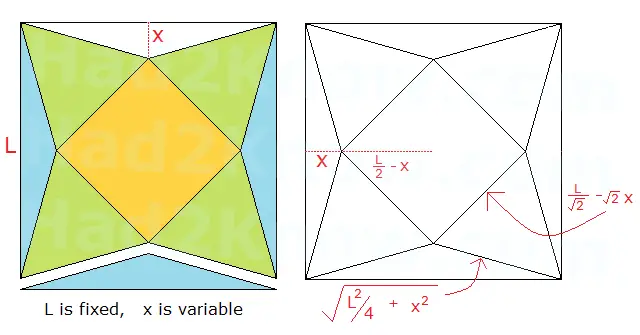# Folding Square Pyramid of Maximal Volume from a Square Piece of Paper

You can construct a pyramid with a square base from a square sheet of paper by centering a pyramid pattern in the middle of the sheet, cutting away the excess, and then sealing the triangular edges together. The figure below shows how a square pyramid is constructed from a square with side lengths of L. The base is centered diagonally in the center of the square, the green triangles form the sides of the pyramid, and the blue triangles on the outside are cut away. Depending on how large you make the blue triangles, you will end up with pyramids of varying shapes and sizes. You can use calculus to determine how large the blue triangles should be so as to maximize the volume of the pyramid.Assuming that L is fixed, the variable responsible for creating pyramids of different sizes is X, the height of the blue triangles. We can use a little geometry to find the volume of the pyramid in terms of L and X.

First, we use the fact that the volume of a pyramid is (1/3)B2H, where B is the length of the base and H is the height of the pyramid. Using a few applications of the Pythagorean theorem, we can see that

B = L/sqrt(2) - sqrt(2)X
H2 = [sqrt(L2/4 + x2)]2 - (L/2 - X)2
H = sqrt(LX)

So the volume is given by the equation

V = (1/3)(sqrt(LX))(L/sqrt(2) - sqrt(2)X)2

By taking the derivative of V and solving dV/dX = 0, we will get the optimal value of X:

V = [0.5L5/2X1/2 - 2L3/2X3/2 + 2L1/2X5/2]/3
dV/dX = [0.25L5/2X-1/2 - 3L3/2X1/2 + 5L1/2X3/2]/3

[0.25L5/2X-1/2 - 3L3/2X1/2 + 5L1/2X3/2]/3 = 0
0.25L2 - 3LX + 5X2 = 0
X = [3L ± sqrt(9L2 - 5L2)/10
X = L/10 and L/2

The solution X = L/2 corresponds to a pyramid with no volume, therefore, the unique solution is L/10. This means that in order to construct a square pyramid with the largest possible volume, you should make X equal to one tenth of the side length.